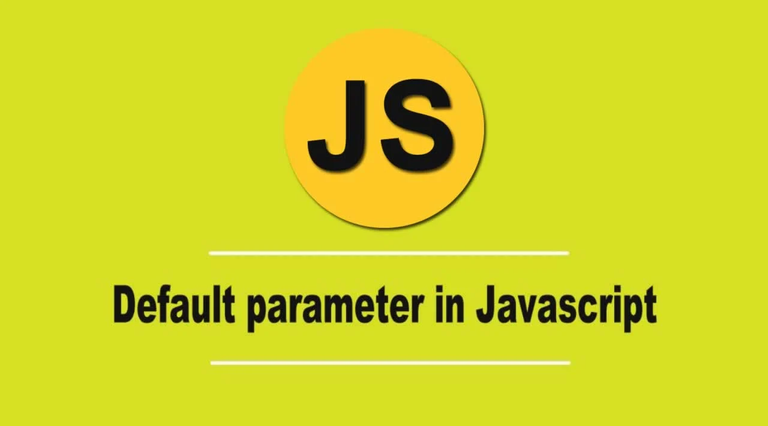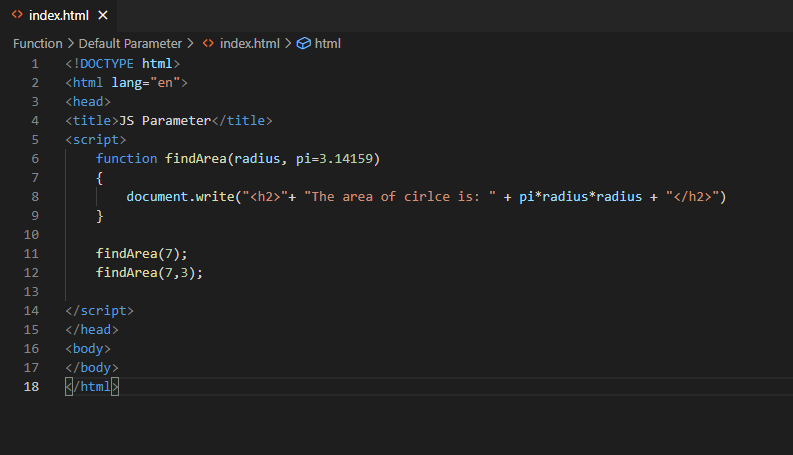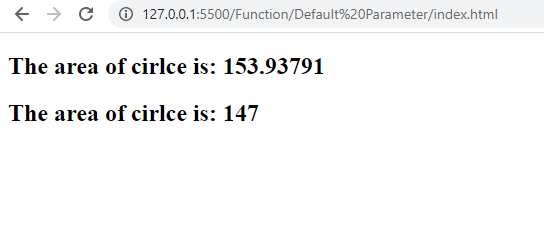# JavaScript Default and Optional Function Parameter

in STEMGeeks2 years agoImage Source

In JAvaScript function, you can pass a default parameter value to the function and to do so such default parameter value should always be at the end inside the parenthesis. While calling the function you don't need to pass the value for default parameter and even if you pass the value, then this new value will override the default parameter value.

In the following code, we will see a simple example of finding the area of the circle.

``````<!DOCTYPE html>
<html lang="en">
<title>JS Parameter</title>
<script>
{
}

findArea(7);
findArea(7,3);

</script>
<body>
</body>
</html>
``````

The same code in my VS Code:You can see we have passed default value of π(pi) as 3.14159 as function parameter. In line number 11, we wanted to find the area of circle with radius 7. So the answer will be (3.1415977=153.93791). In the same way we wanted to find the area of circle with same radius but this time we are overriding the default value of parameter pi to be 3. So it will give answer as: (377=147). Here's the ouput if you run the above code in the browser:There's also another slight similiar concept called optional parameter in JavaScript that works similar to above. I don't seem to find any difference between optional and default parameter. I found both of them have same working process beside the syntax difference. The optional parameter can be defined using the Logical OR operator.

``````function findArea(radius, pi)
{
pi = pi || 3.14159;